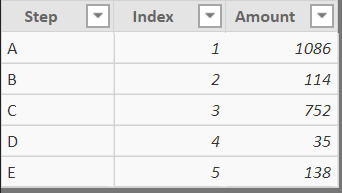2 views
in BI

I am looking for help calculating the difference between the previous row, and the % after that in order to build a funnel in Power Bi.Could anyone help me?

by (47.2k points)
• Use the following table to prototype as a solution:

• Table name: "Data"• column names are slightly different, to make measure naming easier; change them as you prefer.

• Create first measure:

• Total Amount = SUM(Data[Amount])

• Create second measure:

• Accumulated Difference = VAR Current_Index = MAX ( Data[Index] ) VAR Initial_Amount = CALCULATE ( MAX ( Data[Amount] ), FILTER ( ALL ( Data ), Data[Index] = 1 ) ) VAR Accumulated_Reductions = CALCULATE ( [Total Amount], FILTER ( ALL ( Data ), Data[Index] > 1 && Data[Index] <= Current_Index ) ) RETURN Initial_Amount - Accumulated_Reductions

• Create third measure:

• % Previous = VAR Current_Index = MAX ( Data[Index] ) VAR Current_Difference = CALCULATE ( [Accumulated Difference], FILTER ( ALL ( Data ), Data[Index] = Current_Index ) ) VAR Previous_Difference = IF (Current_Index > 1, CALCULATE ( [Accumulated Difference], FILTER ( ALL ( Data ), Data[Index] = Current_Index - 1 ) ) ) RETURN DIVIDE ( Current_Difference, Previous_Difference )

Result:Explanation:

• First measure is simply for convenience, to avoid writing the same summation multiple times.

• Second measure: First, we find index visible in a current row and save it in a variable. Then, we find the initial amount (amount where index = 1), because we need it as a starting point. ALL is necessary to ignore filters applied to the row. Then, using a similar pattern, we calculate accumulated reductions between current and the initial rows (for example, for step D, we need to sum amounts for indexes (4, 3, 2)) Finally, the desired result is simply initial amount - accumulated reductions.

• Third measure: Using very similar technique, we first find Accumulated Difference for the current row, then for the previous row. The only nuance here is to test previous row for the starting conditions (if it's the initial row with index 1, don't calculate previous values). Once current and previous differences are known, we simply divide them.

If you are preparing for the Power BI certification exam, then take up this Power BI training by Intellipaat that offers instructor-led training, hands-on projects, and certification.